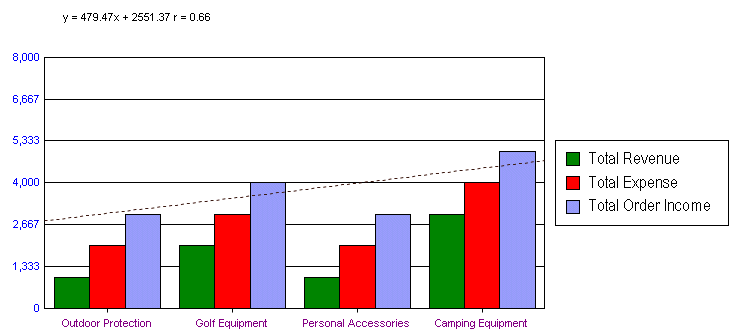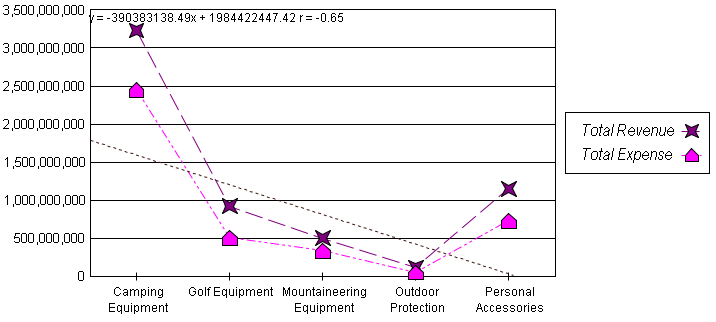# @TRENDLINE_ALLDATA (Linear Regression Line)

This macro draws a linear regression line through all data points in a chart.

SYNTAX:

 `@TRENDLINE_ALLDATA bShowLinearLine bShowEquation`

PARAMETERS:

bShowLinearLine; 1=Show Linear Regression Line, 0=Hide Linear Regression Line

bShowEquation; 1=Show Equation, 1=Hide Equation

EXAMPLE:

 ```@TRENDLINE_ALLDATA 1 1 ``````@TRENDLINE_ALLDATA 1 1 ```PERSISTENT:

YES

ALSO SEE:

@MOVA; to draw a moving average line

@TRENDLINE; to draw a trend line

 ``` ```

Next MacroPrevious MacroReturn to Alpha List

Next Macro in this GroupPrevious Macro in this GroupReturn to Group List